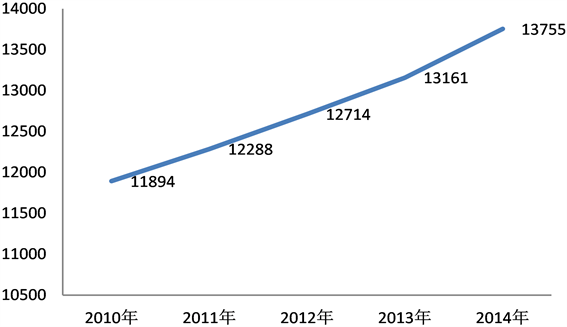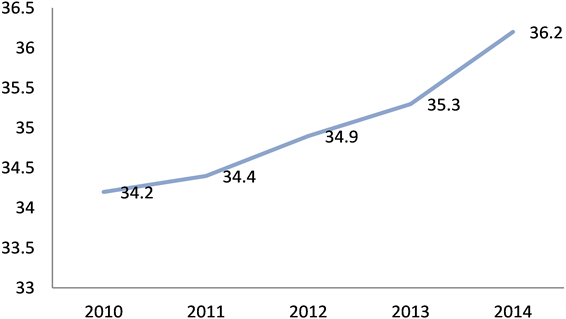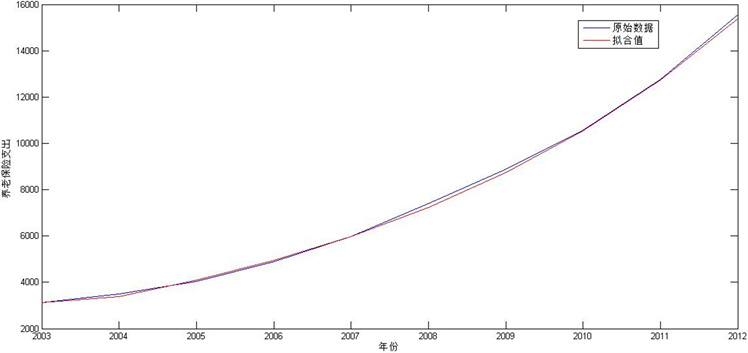﻿ 我国城镇居民养老保险支出预测分析—基于灰色GM(1,1)模型

# 我国城镇居民养老保险支出预测分析—基于灰色GM(1,1)模型Forecast Analysis of Urban Residents’ Endowment Insurance Expenditure in China—Based on Grey GM(1,1) Model

Abstract: With the development of science and technology and medical technology, people’s overall life ex-pectancy is prolonged, so the pension fund has brought great challenges. Therefore, the precise forecast and analysis of the endowment insurance for urban residents in China can lay foundation for the follow-up study of the endowment insurance system in the future. Based on the data of the elderly population and the dependency rate from 2010 to 2014, this paper firstly makes a descriptive analysis of the overall elderly population in China; secondly, it uses the grey GM(1,1) model to forecast and analyze the residents’ pension insurance expenditure. The results show that: China has shown an aging trend; pension expenditure is showing an upward trend year by year; therefore, the government should adjust and improve the relevant policies.

1. 引言

2. 我国65岁及以上人口变化趋势的描述统计分析

3. 灰色预测基本理论及模型介绍

3.1. GM(1,1)模型建立 

$\left({x}^{\left(0\right)}\left(1\right),{x}^{\left(0\right)}\left(2\right),\cdots ,{x}^{\left(0\right)}\left(M\right)\right)$ 是所要预测的某项指标的原始数据。原式数据一次累加：

${x}^{\left(1\right)}\left(k\right)=\underset{i=1}{\overset{k}{\sum }}{x}^{\left(0\right)}\left(t\right),\text{\hspace{0.17em}}\text{\hspace{0.17em}}k=1,2,\cdots ,n$ (1)

${Z}^{\left(1\right)}$${X}^{\left(1\right)}$ 的紧邻均值生成序列，即：Figure 1. Total population aged 65 and over in China from 2010 to 2014Figure 2. China’s old-age dependency ratio from 2010 to 2014

${z}^{\left(1\right)}\left(k\right)=0.5{x}^{\left(1\right)}\left(k\right)+0.5{x}^{\left(1\right)}\left(k-1\right)$ (2)

${Z}^{\left(1\right)}=\left({z}^{\left(1\right)}\left(2\right),{z}^{\left(1\right)}\left(3\right),\cdots ,{z}^{\left(1\right)}\left(n\right)\right)$ (3)

${x}^{\left(0\right)}\left(k\right)+a{z}^{\left(1\right)}\left(k\right)=b$ (4)

$\stackrel{^}{\alpha }={\left({B}^{\text{T}}B\right)}^{-1}{B}^{\text{T}}{Y}_{n}$ (5)

$B=\left[\begin{array}{cc}-{z}^{\left(1\right)}\left(2\right)& 1\\ -{z}^{\left(1\right)}\left(3\right)& 1\\ ⋮& ⋮\\ -{z}^{\left(1\right)}\left(n\right)& 1\end{array}\right],\text{\hspace{0.17em}}\text{\hspace{0.17em}}{Y}_{n}=\left[\begin{array}{c}{x}^{\left(0\right)}\left(2\right)\\ {x}^{\left(0\right)}\left(3\right)\\ ⋮\\ {x}^{\left(0\right)}\left(n\right)\end{array}\right]$ (6)

$\frac{\text{d}{x}^{\left(1\right)}}{\text{d}t}+a{x}^{\left(1\right)}=b$ (7)

${\stackrel{^}{x}}^{\left(1\right)}\left(t\right)=\left({x}^{\left(1\right)}\left(0\right)-\frac{b}{a}\right){\text{e}}^{-at}+\frac{b}{a}$ (8)

GM(1,1)灰色微分方程的时间响应序列为：

${\stackrel{^}{x}}^{\left(1\right)}\left(k+1\right)=|{x}^{\left(1\right)}\left(0\right)-\frac{b}{a}|{\text{e}}^{-ak}+\frac{b}{a},\text{\hspace{0.17em}}k=1,2,\cdots ,n$ (9)

${x}^{\left(1\right)}\left(0\right)={x}^{\left(0\right)}\left(1\right)$ ，则

${\stackrel{^}{x}}^{\left(1\right)}\left(k+1\right)=|{x}^{\left(0\right)}\left(1\right)-\frac{b}{a}|{\text{e}}^{-ak}+\frac{b}{a},k=1,2,\cdots ,n$ (10)

${\stackrel{^}{x}}^{\left(0\right)}\left(k+1\right)={\stackrel{^}{x}}^{\left(1\right)}\left(k+1\right)-{\stackrel{^}{x}}^{\left(1\right)}\left(k\right)$ (11)

3.2. GM(1,1)模型拟合检验

3.2.1. 残差检验

$\stackrel{¯}{e}=\frac{\sum |\stackrel{^}{x\left(t\right)}-x\left(t\right)|}{\sum x\left(t\right)}$ (12)

3.2.2. 关联度检验

${\xi }_{i}=\frac{{\Delta }_{\mathrm{min}}+{\Delta }_{\mathrm{max}}}{{\Delta }_{i}+{\Delta }_{\mathrm{max}}},t=1,2,\cdots ,n$ (13)

3.2.3. 后验差检验

$C=\frac{{S}_{d}}{{S}_{x}}$ (14)

$P=P\left\{|{\Delta }^{\left(0\right)}\left(i\right)-{\stackrel{¯}{\Delta }}^{\left(0\right)}|<0.6745{S}_{x}\right\}$ (15)

4. 我国城镇居民养老保险支出灰色预测模型的建立

4.1. 预测模型的建立

${x}^{0}\left(t\right)=\left\{3122.1,3502.1,4040.3,4896.7,5964.9,7389.6,8894.4,10554.9,12764.9,15561.8\right\}$ 由(1)式得一次累加数据序列：

${x}^{1}\left(t\right)=\left\{3122,6624,10664,15561,21526,28916,37810,48365,61130,76692\right\}$

${B}^{\text{T}}=\left[\begin{array}{ccccccccc}-4873& -8644& -13113& -18544& -25221& -33363& -43088& -54747& -68911\\ 1& 1& 1& 1& 1& 1& 1& 1& 1\end{array}\right]$

$Y=\left\{3502,4040,4897,5965,7390,8894,10555,12765,15562\right\}$

$\left[\begin{array}{c}a\\ b\end{array}\right]=\left[\begin{array}{c}-0.1889\\ 2495.6\end{array}\right]$

${\stackrel{^}{x}}^{\left(1\right)}\left(k+1\right)=16333.32{\text{e}}^{0.1889k}-13211.2$ (16)

${\stackrel{^}{x}}^{\left(0\right)}\left(k+1\right)=\left(1-{\text{e}}^{a}\right)\left({x}^{\left(0\right)}\left(1\right)-\frac{b}{a}\right){\text{e}}^{-ak}$ ，其中 $k=1,2，\cdots ,n$ (17)Table 1. Pension insurance expenditures

${\stackrel{^}{x}}^{\left(0\right)}\left(k+1\right)=2811.4625{\text{e}}^{0.1889k}$ (18)

4.2. 预测模型的检验

4.2.1. 残差检验

4.2.2. 关联度检验

4.2.3. 后验差检验

4.3. 我国城镇居民养老保险支出额GM(1,1)模型预测

5. 总结Table 2. Relative error between calculated and actual value of urban residents’ pension insurance expenditure in ChinaFigure 3. China’s pension insurance expenditures from 2003 to 2012Table 3. Forecast of China’s urban residents’ pension insurance expenditure

 周瑞平. GM(1,1)模型灰色预测法预测城市人口规模[J]. 旅游论坛, 2005, 34(1): 81-83.

 王瑞娜, 唐德善. 基于改进的灰色GM(1,1)模型的人口预测[J]. 统计观测, 2007, 2(20): 93-95.

 赖红松, 祝国瑞, 董品杰. 基于灰色预测和神经网络的人口预测[J]. 经济地理, 2004, 24(2): 198-201.

 白先春, 李炳俊. 基于新陈代谢GM(1,1)模型的我国人口城市化水平分析[J]. 统计与决策, 2006(3): 40-41.

 朱明清, 王智灵, 陈宗海. 基于灰色预测模型和粒子滤波的视觉目标跟踪算法[J]. 控制与决策, 2012, 27(1): 53-57.

Top